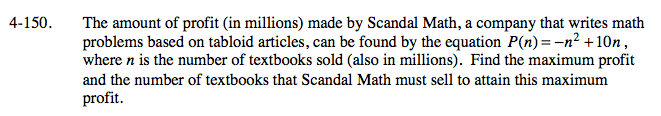### Home > A2C > Chapter Ch4 > Lesson 4.3.2 > Problem4-150

4-150.

The amount of profit (in millions) made by Scandal Math, a company that writes math problems based on tabloid articles, can be found by the equation P(n) = −n2 + 10n, where n is the number of textbooks sold (also in millions). Find the maximum profit and the number of textbooks that Scandal Math must sell to attain this maximum profit. Homework Help ✎To find the maximum, you need to find the vertex of the parabola. Write the equation in graphing form so you can find the vertex.

It is easier to complete the square without the −n2. Start by multiplying both sides by −1 so −P(n) = n2 − 10n. Now complete the square.

Do not forget to multiply again by −1 after you complete the square.

The vertex is your maximum y-value.